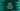# Learn default arguments in Kotlin functions with example# Default arguments in Kotlin functions :

This post is about “default arguments” in kotlin and their use cases.

Default arguments, as the name suggests, are parameters with default values in functions. This is used to mainly reduce the number of overloads. Default arguments are used if we don’t pass any argument. Let’s try to understand how default arguments work with examples :

## Default arguments example :

Let’s try to understand with one example :

``````import java.util.Arrays

fun main(args: Array) {
printValues(1,2)
}

fun printValues(first : Int = 10, second : Int = 20){
println(first)
println(second)
}``````

Now, let’s change the program as below :

``````import java.util.Arrays

fun main(args: Array) {
printValues(1)
}

fun printValues(first : Int = 10, second : Int = 20){
println(first)
println(second)
}``````

Here, we are passing only one argument 1 to the function. But if you run the program, it will print 1 and 20 as the output. So, as you can see that the first element is the same. But the second element is the default one used in the function. We were not passing the second argument, it took the default one.

If we use the above example with a named argument like below :

``````import java.util.Arrays

fun main(args: Array) {
printValues(second = 1)
}

fun printValues(first : Int = 10, second : Int = 20){
println(first)
println(second)
}``````

It will print the first argument default value.

If we don’t pass any argument, it will print the default values :

``````import java.util.Arrays

fun main(args: Array) {
printValues()
}

fun printValues(first : Int = 10, second : Int = 20){
println(first)
println(second)
}``````

## Default values for overridden methods :

If a method is overriding another method, it will use the same default parameter as the parent method. For example, the below function :

``````import java.util.Arrays

fun main(args: Array) {
val b = B()
b.printValues()
}

open class A {
open fun printValues(first : Int = 10, second : Int = 20){
}
}

class B : A(){
override fun printValues(first: Int, second : Int){
println(first)
println(second)
}
}``````

It will print 10 and 20 for the first and second inside the overridden method of class B. If we try to change the default value of first or second in this method, it will throw one error: “error: an overriding function is not allowed to specify default values for its parameters”.

You might also like :